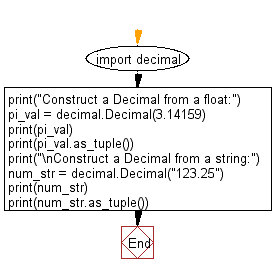﻿ Python: Construct a Decimal from a float and a Decimal from a string - w3resource# Python: Construct a Decimal from a float and a Decimal from a string

## Python module: Exercise-1 with Solution

Write a Python program to construct a Decimal from a float and a Decimal from a string. Also represent the Decimal value as a tuple. Use decimal.Decimal

Sample Solution:

Python Code:

``````import decimal
print("Construct a Decimal from a float:")
pi_val = decimal.Decimal(3.14159)
print(pi_val)
print(pi_val.as_tuple())
print("\nConstruct a Decimal from a string:")
num_str = decimal.Decimal("123.25")
print(num_str)
print(num_str.as_tuple())
```
```

Sample Output:

```Construct a Decimal from a float:
3.14158999999999988261834005243144929409027099609375
DecimalTuple(sign=0, digits=(3, 1, 4, 1, 5, 8, 9, 9, 9, 9, 9, 9, 9, 9, 9, 9, 8, 8, 2, 6, 1, 8, 3, 4, 0, 0, 5, 2, 4, 3, 1, 4, 4, 9, 2, 9, 4, 0, 9, 0, 2, 7, 0, 9, 9, 6, 0, 9, 3, 7, 5), exponent=-50)

Construct a Decimal from a string:
123.25
DecimalTuple(sign=0, digits=(1, 2, 3, 2, 5), exponent=-2)
```

Flowchart:## Visualize Python code execution:

The following tool visualize what the computer is doing step-by-step as it executes the said program:

Python Code Editor:

Have another way to solve this solution? Contribute your code (and comments) through Disqus.

What is the difficulty level of this exercise?

Test your Python skills with w3resource's quiz

﻿

## Python: Tips of the Day

Dictionary comprehension:

```>>> m = {x: x ** 2 for x in range(5)}
>>> m
{0: 0, 1: 1, 2: 4, 3: 9, 4: 16}
```